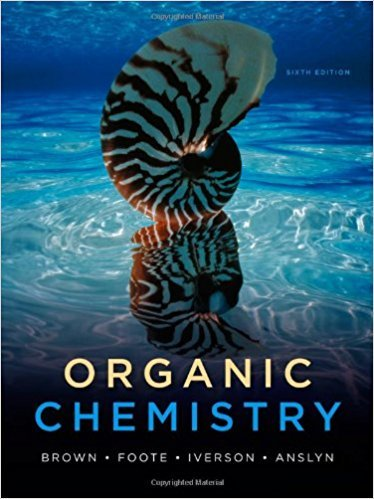×

# Solutions for Chapter 28: Organic Chemistry 6th Edition## Full solutions for Organic Chemistry | 6th Edition

ISBN: 9780840054982Solutions for Chapter 28

Solutions for Chapter 28
4 5 0 407 Reviews
29
4
##### ISBN: 9780840054982

This textbook survival guide was created for the textbook: Organic Chemistry, edition: 6. Since 38 problems in chapter 28 have been answered, more than 24640 students have viewed full step-by-step solutions from this chapter. Chapter 28 includes 38 full step-by-step solutions. This expansive textbook survival guide covers the following chapters and their solutions. Organic Chemistry was written by and is associated to the ISBN: 9780840054982.

Key Chemistry Terms and definitions covered in this textbook
• alkane

A hydrocarbon that lacks p bonds.

• Alkene metathesis

A reaction in which two alkenes interchange the carbons attached to their double bonds.

• binary compounds.

Compounds formed from just two elements. (2.7)

• column chromatography

A technique by which compounds are separated from each other based on a difference in the way they interact with the medium (the adsorbent) through which they are passed.

• Coulomb’s law.

The potential energy between two ions is directly proportional to the product of their charges and inversely proportional to the distance between them. (9.3)

Reactions in which two p systems are joined together in a way that forms a ring. In the process, two p bonds are converted into two s bonds.

• electron capture

A mode of radioactive decay in which an inner-shell orbital electron is captured by the nucleus. (Section 21.1)

• Enthalpy change, DH

The difference in total bond strengths and solvation between various points under comparison on a reaction coordinate diagram

• equilibrium-constant expression

The expression that describes the relationship among the concentrations (or partial pressures) of the substances present in a system at equilibrium. The numerator is obtained by multiplying the concentrations of the substances on the product side of the equation, each raised to a power equal to its coefficient in the chemical equation. The denominator similarly contains the concentrations of the substances on the reactant side of the equation. (Section 15.2)

• heterocycle

A cyclic compound containing at least one heteroatom (such as S, N, or O) in the ring.

• Lewis structures

A drawing style inwhich the electrons take center stage.linear polymer (Sect. 27.6): A polymer thathas only a minimal amount of branching or nobranching at all.

• miscible liquids

Liquids that mix in all proportions. (Section 13.3)

• pi (p) bond

A bond formed from adjacent, overlapping p orbitals.

• Polarizability

A measure of the ease of distortion of the distribution of electron density about an atom or group in response to interaction with other molecules or ions. Fluorine which has a high electronegativity and holds its electrons tightly, has a very low polarizability. Iodine, which has a lower electronegativity and holds its electrons less tightly, has a very high polarizability.

• Pro-R-hydrogen

Replacing this hydrogen by deuterium gives a chiral center with an R confi guration

• proton

A positively charged subatomic particle found in the nucleus of an atom. (Section 2.3)

• R,S System

A set of rules for specifying absolute confi guration about a chiral center; also called the Cahn-Ingold-Prelog system

• rate equation

An equation thatdescribes the relationship between the rate of a reactionand the concentration of reactants.

• weak activators

Groups that weakly activate an aromatic ring toward electrophilic aromatic substitution, thereby enhancing the rate of the reaction.

• Z

For alkenes, a stereodescriptor that indicates that the two priority groups are on the same side of the p bond.

×